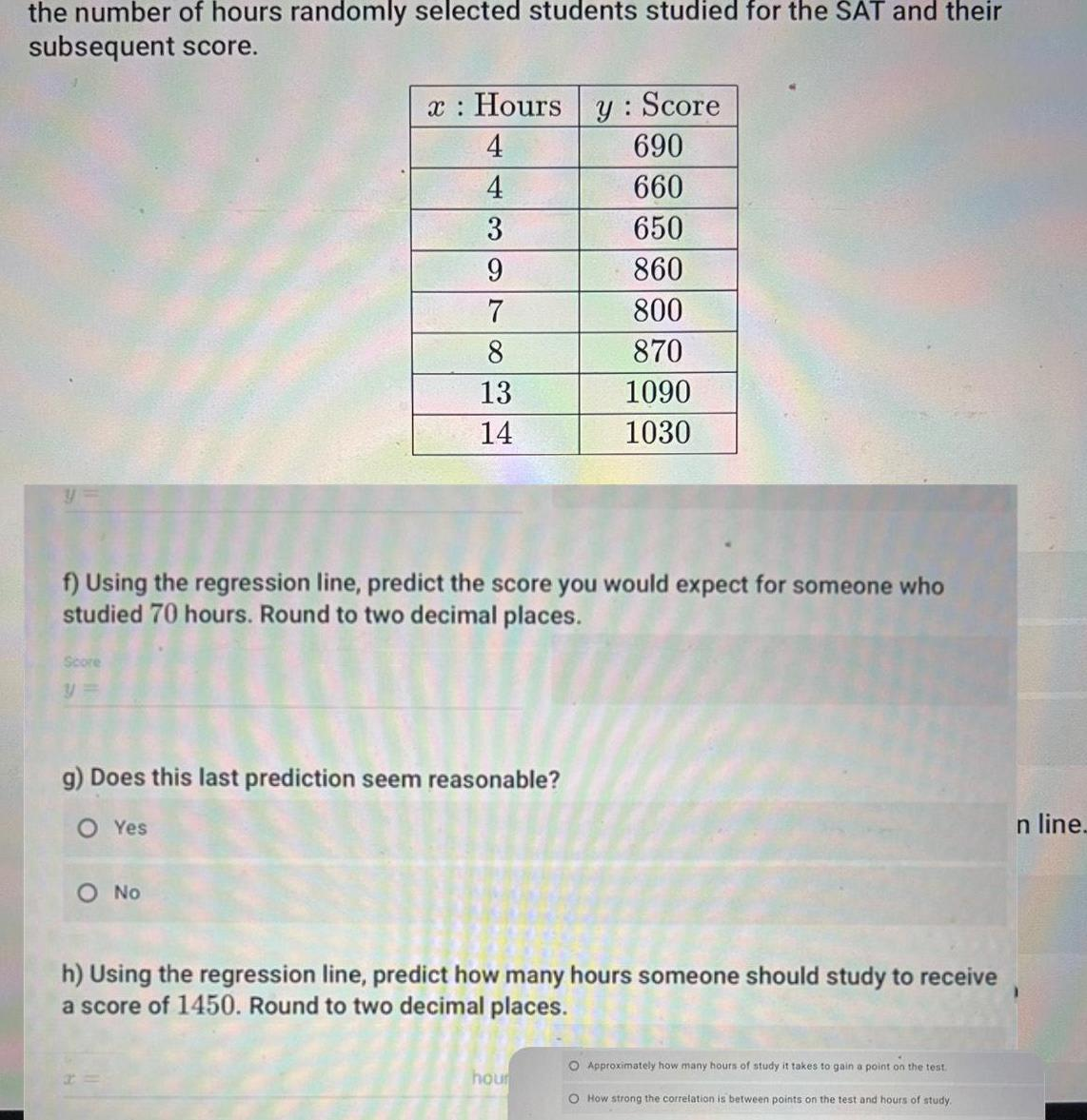Question:

# the number of hours randomly selected students studied for

Last updated: 9/18/2023the number of hours randomly selected students studied for the SAT and their subsequent score Score y x Hours 4 4 3 9 7 8 13 14 f Using the regression line predict the score you would expect for someone who studied 70 hours Round to two decimal places g Does this last prediction seem reasonable O Yes O No y Score 690 660 650 860 800 870 1090 1030 h Using the regression line predict how many hours someone should study to receive a score of 1450 Round to two decimal places hour O Approximately how many hours of study it takes to gain a point on the test O How strong the correlation is between points on the test and hours of study n line